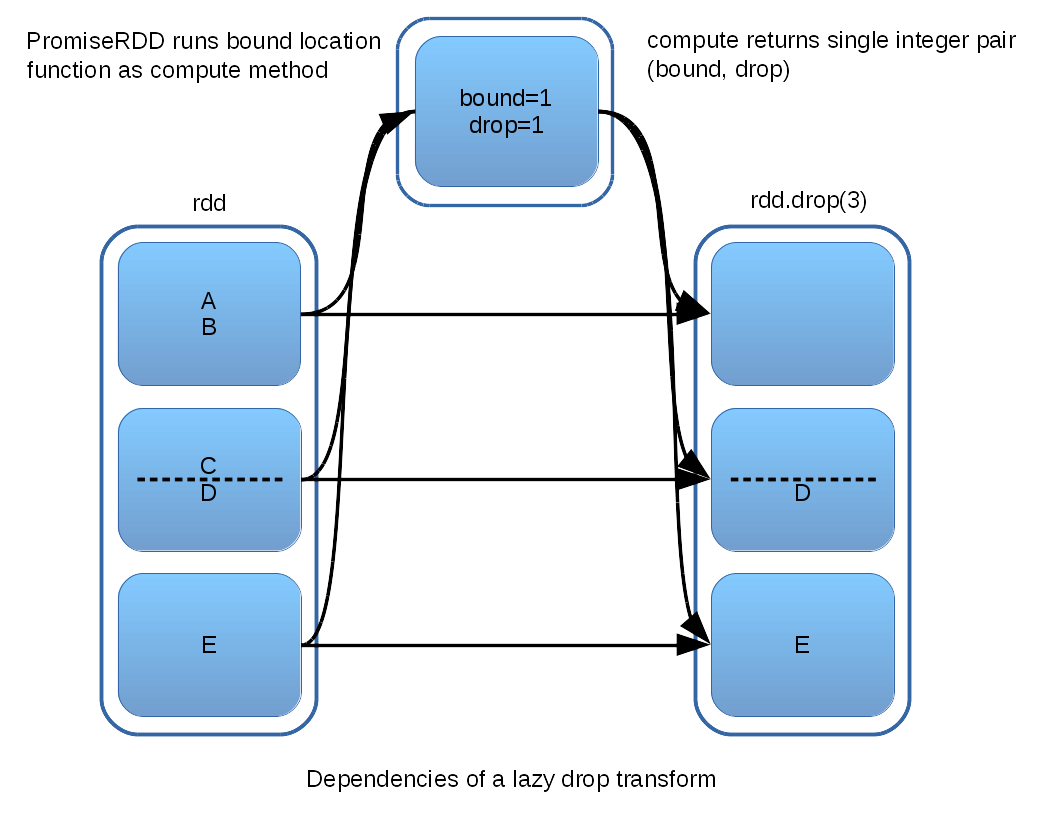In a previous post I described a method for implementing the Scala drop transform for Spark RDDs. That implementation came at a cost of subverting the RDD lazy transform model; it forced the computation of one or more input RDD partitions at call time instead of deferring partition computation, and so behaved more like a Spark action than a transform.

In this followup post I will describe how to implement drop as a true lazy RDD transform, using a new RDD subclass: the Promise RDD. A Promise RDD can be used to embed computations in the lazy transform formalism that otherwise would require non-lazy actions.

The Promise RDD (aka PromiseRDD subclass) is designed to encapsulate a single expression value in an RDD having exactly one row, to be evaluated only if and when its single partition is computed. It behaves somewhat analogously to a Scala promise structure, as it abstracts the expression such that any requests for its value (and hence its actual computation) may be deferred.

The definition of PromiseRDD is compact:

class PromisePartition extends Partition {
// A PromiseRDD has exactly one partition, by construction:
override def index = 0
}

/**
* A way to represent the concept of a promised expression as an RDD, so that it
* can operate naturally inside the lazy-transform formalism
*/
class PromiseRDD[V: ClassTag](expr: => (TaskContext => V),
context: SparkContext, deps: Seq[Dependency[_]])
extends RDD[V](context, deps) {

// This RDD has exactly one partition by definition, since it will contain
// a single row holding the 'promised' result of evaluating 'expr'
override def getPartitions = Array(new PromisePartition)

// compute evaluates 'expr', yielding an iterator over a sequence of length 1:
override def compute(p: Partition, ctx: TaskContext) = List(expr(ctx)).iterator
}


A PromiseRDD is constructed with the expression of choice, embodied as a function from a TaskContext to the implied expression type. Note that only the task context is a parameter; Any other inputs needed to evaluate the expression must be present in the closure of expr. This allows the expression to be of very general form: its value may depend on a single input RDD, or multiple RDDs, or no RDDs at all. It receives an arbitrary sequence of partition dependencies which is the responsibility of the calling code to assemble. Again, this allows substantial generality in the form of the expression: the PromiseRDD dependencies can correspond to any arbitrary input dependencies assumed by the expression. The dependencies can be tuned to exactly what input partitions are required.

As a motivating example, consider how a PromiseRDD can be used to promote drop to a true lazy transform. The aspect of computing drop that threatens laziness is the necessity of determining the location of the boundary partition (see previous discussion). However, this portion of the computation can in fact be encapsulated in a PromiseRDD. The details of constructing such a PromiseRDD can be viewed here. The following illustration summarizes the topology of the dependency DAG that is constructed:As the dependency diagram shows, the PromiseRDD responsible for locating the boundary partition depends on each partition of the original input RDD. The actual computation is likely to only request the first input partition, but all partitions might be required to handle all possible arguments to drop. In turn, the location information given by the PromiseRDD is depended upon by each output partition. Input partitions are either passed to the output, or used to compute the boundary, and so none of the partition computation is wasted.

Observe that the scheduler remains in charge of when partitions are computed. An advantage to using a PromiseRDD is that it works within Spark’s computational model, instead of forcing it.

The following brief example demonstrates that drop implemented using a PromiseRDD satisfies the lazy transform model:

// create data rdd with values 0 thru 9
scala> val data = sc.parallelize(0 until 10)
data: org.apache.spark.rdd.RDD[Int] = ParallelCollectionRDD at parallelize at <console>:12

// drop the first 3 rows
// note that no action is performed -- this transform is lazy
scala> val rdd = data.drop(3)
rdd: org.apache.spark.rdd.RDD[Int] = $anon$1 at drop at <console>:14

// collect the values.  This action kicks off job scheduling and execution
scala> rdd.collect
14/07/28 12:16:13 INFO SparkContext: Starting job: collect at <console>:17
... job scheduling and execution output ...

res0: Array[Int] = Array(3, 4, 5, 6, 7, 8, 9)

scala>


In this post, I have described the Promise RDD, an RDD subclass that can be used to encapsulate computations in the lazy transform formalism that would otherwise require non-lazy actions. As an example, I have outlined a lazy transform implementation of drop that uses PromiseRDD.